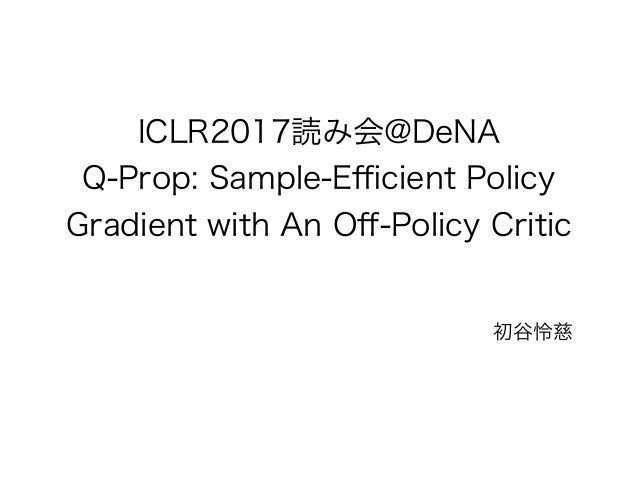Successfully reported this slideshow.
We use your LinkedIn profile and activity data to personalize ads and to show you more relevant ads. You can change your ad preferences anytime.Upcoming SlideShare
Loading in …5
×

# Q prop

26,947 views

Published on

Summary of policy gradient and value gradient with Q-Prop

Published in: Engineering
• Full Name
Comment goes here.

Are you sure you want to Yes No
Your message goes here• Be the first to comment

### Q prop

1. 1. St+1 ~ P( ′s | St ,At ) rt+1 = r(St ,At ,St+1) At ~ π( ′a | St )
2. 2. St+1 ~ P( ′s | St ,At ) rt+1 = r(St ,At ,St+1) At ~ π( ′a | St ) π∗ = argmax π Eπ [ γ τ rτ ] τ =0 ∞ ∑
3. 3. π∗ = argmax π Eπ [ γ τ rτ ] τ =0 ∞ ∑ = J ∇θ J
4. 4. ∇θ J = Eπθ [∇θ log(πθ (at | st ))Qt ] ∇θ J = Es∼ρ ∇aQµ s,a( )a=µθ s( ) ∇θ µθ s( )⎡ ⎣⎢ ⎤ ⎦⎥
5. 5. ∇θ J = ∇θ Eπθ [ γ τ rτ ] τ =0 ∞ ∑ = ∇θ Es0 ~ρ,s'~p πθ at ,st( ) γ τ rτ τ =0 ∞ ∑t=0 ∏ ⎡ ⎣ ⎢ ⎤ ⎦ ⎥ = Es0 ~ρ,s'~p ∇θ πθ at ,st( ) γ τ rτ τ =0 ∞ ∑t=0 ∏ ⎡ ⎣ ⎢ ⎤ ⎦ ⎥ = Es~ρ πθ at ,st( ) ∇θ πθ at ,st( ) t=0 ∏ πθ at ,st( ) t=0 ∏ γ τ rτ τ =0 ∞ ∑ t=0 ∏ ⎡ ⎣ ⎢ ⎢ ⎢ ⎤ ⎦ ⎥ ⎥ ⎥ = Es~ρ πθ (at | st ) ∇θ log(πθ (at | st )) t=0 ∑t=0 ∏ γ τ rτ τ =0 ∞ ∑ ⎡ ⎣ ⎢ ⎤ ⎦ ⎥ = Eπθ [ ∇θ log(πθ (at | st )) t=0 ∑ γ τ rτ τ =t ∞ ∑ ]
6. 6. ∇log p x( )( ) f x( )
7. 7. ∇log p x( )( ) f x( )
8. 8. J = Es∼ρ [Qµθ s,µθ s( )( )] ∇θ J = Es∼ρ ∇θQµ s,µθ s( )( )⎡⎣ ⎤⎦ = Es∼ρ ∇aQµ s,a( )a=µθ s( ) ∇θ µθ s( )⎡ ⎣⎢ ⎤ ⎦⎥
9. 9. f st ,at( )= f st ,at( )+ ∇a f st ,a( )a=at at − at( ) ∇θ J = Eρ,π ∇θ logπθ at st( ) Q st ,at( )− f st ,at( )( )⎡ ⎣ ⎤ ⎦ + Eρ,π ∇θ logπθ at st( ) f st ,at( )⎡ ⎣ ⎤ ⎦ = Eρ,π ∇θ logπθ at st( ) Q st ,at( )− f st ,at( )( )⎡ ⎣ ⎤ ⎦ + Eρ,π ∇a f st ,a( )a=at ∇θ µθ st( )⎡ ⎣ ⎤ ⎦
10. 10. ∇θ J = Eρ,π ∇θ logπθ at st( ) Q st ,at( )−Qw st ,at( )( )⎡ ⎣ ⎤ ⎦ + Eρ,π ∇aQw st ,a( )a=at ∇θ µθ st( )⎡ ⎣ ⎤ ⎦ ∇θ J = Eρ,π ∇θ logπθ at st( ) A st ,at( )− Aw st ,at( )( )⎡ ⎣ ⎤ ⎦ + Eρ,π ∇aQw st ,a( )a=at ∇θ µθ st( )⎡ ⎣ ⎤ ⎦ a
11. 11. ∇θ J = Eρ,π ∇θ logπθ at st( ) A st ,at( )− Aw st ,at( )( )⎡ ⎣ ⎤ ⎦ + Eρ,π ∇aQw st ,a( )a=at ∇θ µθ st( )⎡ ⎣ ⎤ ⎦ Aw = Qw st ,at( )− Eπ Qw st ,at( )⎡⎣ ⎤⎦ = Qw st ,µθ st( )( )+ ∇aQw st ,a( )a=µθ st( ) at − µθ st( )( )− Eπ Qw st ,µθ st( )( )+ ∇aQw st ,a( )a=µθ st( ) at − µθ st( )( )⎡ ⎣⎢ ⎤ ⎦⎥ = ∇aQw st ,a( )a=µθ st( ) at − µθ st( )( ) rt+1 +γV st+1( )−V st( ) Eπ at[ ]= µθ st( )
12. 12. m* = m −η(t −τ ) E m* ⎡⎣ ⎤⎦ = E m[ ] Var m* ⎡⎣ ⎤⎦ = Var m[ ]− 2ηCov m,t[ ]+η2 Var t[ ] η* = Cov m,t[ ] Var t[ ]
13. 13. ∇θ J = Eρ,π ∇θ logπθ at st( ) A st ,at( )−η st( )Aw st ,at( )( )⎡ ⎣ ⎤ ⎦ + Eρ,π η st( )∇aQw st ,a( )a=at ∇θ µθ st( )⎡ ⎣ ⎤ ⎦ Var A −ηAw⎡⎣ ⎤⎦ = Var A[ ]− 2ηCov A,Aw( )+η2 Var Aw( ) η* = Cov A,Aw( ) Var Aw( )